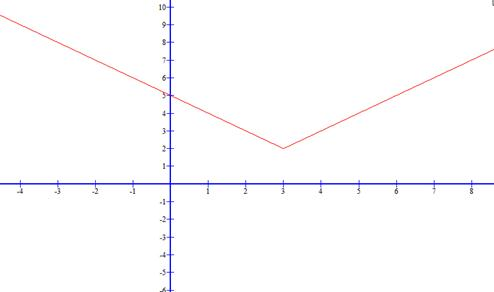### Sample ProblemThis is a graph of the function y=|x-3|+2. Which of the following graphs accurately represents this graph reflected about the x-axis?

#### Solution

The second graph clearly shows that y=|x-3|+2 has been reflected about the x-axis. The last two graphs are reflections about the y-axis and the first graph does not reflect about the x-axis but instead the line y=2. The second graph would have an equation y=-(|x-3|+2) since a reflection in the x-axis is represented as h(x) =-f(x) where y=f(x) is the original function.